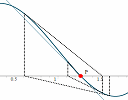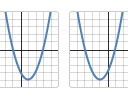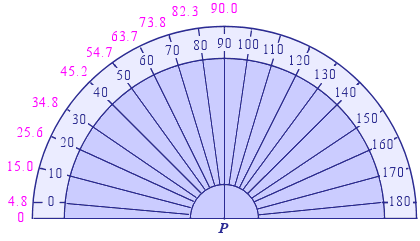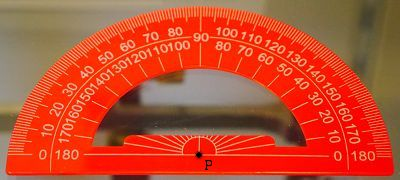Search IntMath
Close

450+ Math Lessons written by Math Professors and Teachers

5 Million+ Students Helped Each Year

1200+ Articles Written by Math Educators and Enthusiasts

Simplifying and Teaching Math for Over 23 Years

# IntMath Newsletter: Newton’s Method, Desmos activities

By Murray Bourne, 30 Jul 2015

30 Jul 2015

1. New interactive graph: Newton's Method
2. Newton's Method, accuracy and floating point numbers
3. Resource: Desmos Activities
4. Math puzzles
5. Math movie: Talks for people who hated math in high school
6. Final thought: Success often means failure first

## 1. New interactive graph: Newton's MethodI added a new interactive graph that helps explain how Newton's Method works. Newton's Method uses differentiation to solve non-linear equations and is especially useful when we can't factor the equation. It's very commonly used by computers when solving math-based problems.

## 2. Newton's Method, accuracy and floating point numbers

While producing the above graph, I came across the usual inaccuracies that arise when floating point numbes are involved.YouTube engineers faced a problem as the number of views for Gangnam Style approached 2 billion. I found related issues when developing the Newton's Method graph. Newton's Method, accuracy and floating point numbers

## 3. Desmos Activities

Desmos is one of the best free online graphing tools. Apart from allowing you to graph functions easily via an intuitive math input system, it's also possible to add sliders to graphs (allowing you to explore the effect of changing parameters). You can graph tables of data and also produce quadratic and exponential regressions.

Desmos is also available on iOS and Android devices.

### The Activities

The teacher-designed math activities provide situations where students can learn math vocabulary and concepts, in a visual context.For example, in the Polygraph activities, one student chooses a specific graph (e.g. a parabola passing through 2 points on the x-axis), and their buddy needs to ask questions to figure out which graph they chose.

It's a great way to engage students by getting them involved in talking about math, and asking questions about math.

Another interesting one is Water Line, where students need to figure out the graph of height versus time for filling various container shapes with water. This is good for developing essential mathematical modeling skills.

## 4. Math puzzles

The puzzle in the last IntMath Newsletter asked what was wrong with two protractors.

Partially correct answers were given by Pavithra, V T, Abhijit, Sudha, Zhuqi and Paul.

Here is a more detailed analysis.

### Both Protractors

The first problem with both of the protractors is they are not transparent. It is possible to make a metal or plastic one without being transparent, but it makes it harder to use. The angle you are measuring has to be at least the length of the radius of the protractor, otherwise you have to extend the arms of the angle. As a manufacturer, you need to decide where to place the origin point. Both protractors had errors.

### The Blue Protractor

In this case, the origin point is placed at the bottom middle of the protractor. This is good in that we can place that point on our angle's vertex. But the and 180° markings should be in line with that origin point. The blue one fails in this respect. The only angle that will be correctly measured is 90°.

I took the photo of the protractor and overlaid it in my image editing software. I then measured the angles as follows.One would expect to be the most inaccurate, then as the angles get bigger, the error should reduce. However, it wasn't even a linear relationship. You can actually see the gap from 3 to 4 is larger than the rest.

All in all, a distrastrous measuring instrument.

### The Red Protractor

The key problem with this protractor is that it's impossible to see the vertex of the angle you are trying to meaure (or construct).

You would need to drill a hole at the point P for it to work properly. Once you've done that, the angles should be reasonably accurate.Of course, the manufacturer should have drilled a hole there. No wonder the students made many mistrakes while using these protractors!

### New math puzzle

Find 3 integers that form a geometric progression if their sum is 21 and the sum of their reciprocals is 7/12.

## 5. Math movies - Talks for people who hated math in high schoolHere's a collection of 6 talks which show mathematics in a different light. We hear from a Web cartoonist, someone who analyzed the likelihood of finding the right mate, a comedian on prime numbers and a performacne of "Mathemagic". I've featured some of these videos before.

## 6. Final thought: Success often means failure first[Image source: Allposters]

I've missed 3000 shots. Twenty-six times the game-winning shot has been trusted to me, and I've missed. I've lost over 300 games. I've failed over and over and over again, and that is why I've succeeded. [Michael Jordan]

Until next time, enjoy whatever you learn.

1. Sundararajan says:

3,6and 12

2. ARUN KUMAR. A says:

required three numbers of gp are 3,6,12

3. ARUN KUMAR. A says:

3, 6, 12

4. Paul G says:

since the sum of the three terms of a geometric series are a(1+r+r^2)= 21 either a =7 or a=3.

If a=3 it means that r=2 so the three integers would be 3, 6 and 12. If a=7 we would have r = 1 which gives 7,7 and 7. Since 1/3 + 1/6 +1/12 = 7/12 the three integers required are 3,6 and12

5. don miller says:

a(r^0)+a(r)+a(r^2) = a(1+r+r^2)= 21

(1/a)+(1/ar)+1/(ar^2) = (r^2 +r+1)/ar^2 = 7/12

sub yields a(r) =6 let a =3, r=2 then

the geometric series becomes

3 + 6 + 12 QED

6. Colin Morrison says:

Answer is geometric progression 3, 6 & 12. Sum 21 and sum of reciprocals 7/12

7. kelvin rosser says:

three integers are 12 6and 3

8. Henry says:

We start with: a + ar + ar.r = 21( a G.P.). Also, 1/a + 1/(ar) + 1/(a.r.r) = 7/12.Rearranging this last one, we get: (r.r)/(a.r.r)+ r/(a.r.r.) + 1/(a.r.r.) = 7/12.

Hence, a.r.r = 12and r.r + r + 1=7. Solving this quadratic, we find that r = 2 or -3; now -3 does not give us an integer for 'a'; so we use r = 2. Thus a.2.2 = 12 and 'a' = 3.

Finally we see that:
3+6+12 = 21 (correct; 1/3 + 1/6 + 1/12 = 7/12 (correct).So the original integers must have been: 3, 6 and 12.

9. tom barrett says:

Let a be the first term and r the common ratio
Then a+ar+ar^2=21 sum =21
So a(1+r+r^2) =21
So 1+r+r^2 = 21/a
or r^2 +r+1 = 21/a

And 1/a + 1/ar +1/ar^2 = 7/12 sum of reciprocals
So (r^2 +r+1)/ar^2 = 7/12
substitute 21/a for r^2 + r +1
(21/a)/ar^2 = 7/12
By algebra we get ar=6
And a = 6/r
now substitute 6/r for a in a +ar+ar^2
And solve the quadratic equation in r
This yields r=2
And a = 3 from ar=6
Result 3,6,12

10. Arthur Simon says:

3,6,12

11. Rutwik Tank says:

Puzzle solution :
3, 6, 12
Explanation :
Let the integers be a, ar, ar^2
So, a(1+r+r^2)=21
and (1/a)((1)+(1/r)+(1/r^2))=7/12
therefore (36/a)((r^2+r+1)/r^2)=21=a+ar+ar^2
therefore (a^2)(r^2)=36
therefore ar=6
Now, a and r are integers.
So, (a,r)=(1,6) or (2,3) or (3,2) or (6,1)
(a,r)=(1,6) is not possible as ar^2 in this case is more than 21.
Let (a,r)=(2,3)
then we get 2,6,18; but 2+6+18 is not equal to 21.
Now let (a,r)=(3,2)
then we get 3,6,12 as required integers.
3+6+12=21 and ((1/3)+(1/6)+(1/12))=7/12

12. hamid zandi says:

6,3,12

13. MAWANDA says:

r/x + x + xr = 21
x/r + 1/x + 1/xr = 7/12

r = (21 - x)/(x + 1/x)

14. Tomas Garza says:

Since a, b and c form a geometric progression, they may be rewritten a, ar and ar^2. Then there are two unknowns, a and r. The conditions are a + ar + ar^2 = 21, and 1/a + 1/ar +1/ar^2 = 7/12. There are four solutions to this pair of equations:

a = 25.5934, r = -0.234436;
a = 12, r = 0.5;
a = 1.40661, r = -4.26556;
a = 3, r = 2.

The explicit values for a, b, c are, respectively,

{25.5934, -6, 1.40661.}
{12, 6, 3}
{1.40661, -6, 25.5934}
{3, 6, 12}

It is a curious fact that the first and the third solutions are the reversion of each other, as well as the second and the fourth.

15. Henry says:

Thanks for the 'Protractor' puzzle! I will use it for my students who are studying maritime subjects and have to do plotting/graph work etc using protractors. It may well make them a little more aware of real problems!! cheers, Henry.

16. Gill says:

3, 6, 12

### Comment Preview

HTML: You can use simple tags like <b>, <a href="...">, etc.

To enter math, you can can either:

1. Use simple calculator-like input in the following format (surround your math in backticks, or qq on tablet or phone):
a^2 = sqrt(b^2 + c^2)
(See more on ASCIIMath syntax); or
2. Use simple LaTeX in the following format. Surround your math with $$ and $$.
$$\int g dx = \sqrt{\frac{a}{b}}$$
(This is standard simple LaTeX.)

NOTE: You can mix both types of math entry in your comment.